Vizard 7 » Reference » Shaders » Post-Process Effects » Blur effects

## Blur effects

The vizfx.postprocess.blur library is a collection of effects that perform various kinds of blurring on the rendered image.

#### Gaussian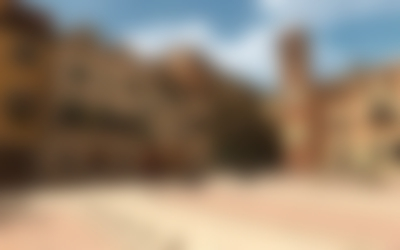Applies a gaussian blur to the image.

 Constructor Description GaussianBlurEffect(     blurRadius = 0,     blurScale = 0.0,     downsample = 0.5 ) Create the gaussian blur effect with the initial blur radius, scale, and downsample values.
 Method Description .setBlurRadius(value) Set the blur radius, in pixels. A higher radius make the image blurrier, but requires more processing time. .getBlurRadius() Get the blur radius, in pixels. .setBlurScale(value) Set the blur scale. Scaling upwards will increase the blurriness without needing extra processing, but at a loss in quality. .getBlurScale() Get the blur scale. This effectively scales the radius, without increasing the number of samples taken. .setDownsample(value) Set the downsample scale factor for the incoming image before performing the blur. Lowering the downsample size will reduce processing time, but at a loss in quality. .getDownsample() Get the downsample scale factor.

This effect can be registered with vizconfig.

import vizfx.postprocess
from vizfx.postprocess.blur import GaussianBlurEffect

#### Box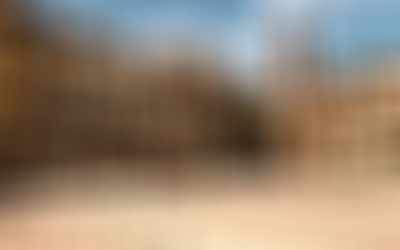Applies a box blur to the image. A box blur is an unweighted blur filter that simply takes the average of the neighboring pixels.

 Constructor Description BoxBlurEffect(     blurRadius = 0,     blurScale = 0.0,     downsample = 0.5 ) Create the gaussian blur effect with the initial blur radius, scale, and downsample values.
 Method Description .setBlurRadius(value) Set the blur radius, in pixels. A higher radius make the image blurrier, but requires more processing time. .getBlurRadius() Get the blur radius, in pixels. .setBlurScale(value) Set the blur scale. Scaling upwards will increase the blurriness without needing extra processing, but at a loss in quality. .getBlurScale() Get the blur scale. This effectively scales the radius, without increasing the number of samples taken. .setDownsample(value) Set the downsample scale factor for the incoming image before performing the blur. Lowering the downsample size will reduce processing time, but at a loss in quality. .getDownsample() Get the downsample scale factor.

This effect can be registered with vizconfig.

import vizfx.postprocess
from vizfx.postprocess.blur import BoxBlurEffect

#### Directional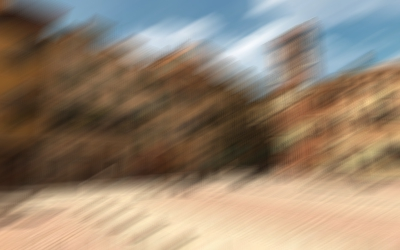Applies a directional blur to the image. All the pixels will be blurred along a single specified direction vector.

 Constructor Description DirectionalBlurEffect(     distance = 0.0,     angle = 0.0,     samples = 20 ) Create the directional blur effect with the initial distance, angle, and blur samples.
 Method Description .setDistance(value) Set the distance along the direction vector to blur, in pixels. .getDistance() Get the distance along the direction vector to blur, in pixels. .setAngle(value) Set the direction angle to blur along, in degrees. 0 is upward, and positive angles go clockwise. .getAngle() Get direction angle to blur along, in degrees. .setSamples(value) Set the number of blur samples per pixel. More samples make the blur smoother, but requires more computation. .getSamples() Get the number of blur samples per pixel.

This effect can be registered with vizconfig.

import vizfx.postprocess
from vizfx.postprocess.blur import DirectionalBlurEffect
effect = DirectionalBlurEffect(angle=60, distance=40)

#### Zoom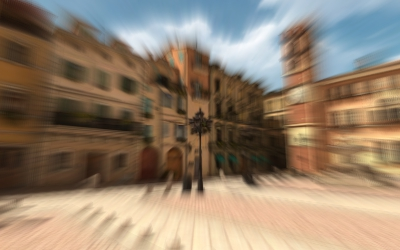Applies a zoom blur to the image. All the pixels will be blurred towards the specified center of the image.

 Constructor Description ZoomBlurEffect(     zoom = 0.0,     radius = 0.0,     center = (0.5,0.5),     samples = 20 ) Create the zoom blur effect with the initial zoom factor, radius, center, and blur samples.
 Method Description .setZoom(value) Set the zoom factor. A positive value zooms in and negative zooms out. .getZoom() Get the zoom factor. .setRadius(value) Set the radius about the center where the blurring will begin. The radius is in normalized (0-1) image units. .getRadius() Get the radius in normalized (0-1) image units. .setCenter(value) Set the zoom [x,y] center in normalized (0-1) image units. .getCenter() Get the zoom [x,y] center in normalized (0-1) image units. .setSamples(value) Set the number of blur samples per pixel. More samples make the blur smoother, but requires more computation. .getSamples() Get the number of blur samples per pixel.

This effect can be registered with vizconfig.

import vizfx.postprocess
from vizfx.postprocess.blur import ZoomBlurEffect
effect = ZoomBlurEffect(zoom=0.2)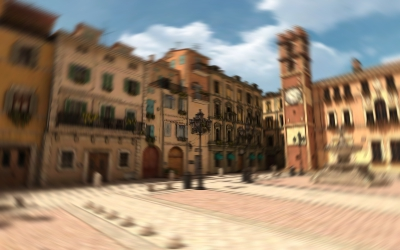Applies a radial blur to the image. All the pixels will be blurred around the specified center of the image.

 Constructor Description RadialBlurEffect(     angle = 0.0,     radius = 0.0,     center = (0.5,0.5),     samples = 20 ) Create the radial blur effect with the initial blur angle, radius, center, and blur samples.

This effect can be registered with vizconfig.

import vizfx.postprocess

#### Fragment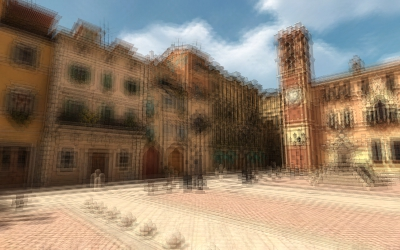Applies a fragment blur to the image. Multiple copies (fragments) of the image are drawn at a specified distance and in equal angular increments from the center.

 Constructor Description FragmentBlurEffect(     distance = 0,     angle = 0.0,     samples = 4 ) Create the fragment blur effect with the initial distance , angle, and fragment samples.
 Method Description .setDistance(value) Set the distance of each fragment in pixels. .getDistance() Get the distance of each fragment in pixels. .setAngle(value) Set rotation angle offset of each fragment, in degrees. .getAngle() Get rotation angle offset of each fragment, in degrees. .setSamples(value) Set the number of blur samples per pixel. More samples make the blur smoother, but requires more computation. .getSamples() Get the number of blur samples per pixel.

This effect can be registered with vizconfig.

import vizfx.postprocess
from vizfx.postprocess.blur import FragmentBlurEffect
effect = FragmentBlurEffect(distance=4)

#### Tilt Shift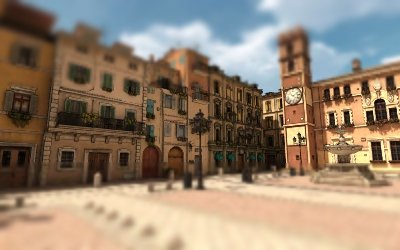Applies a tilt shift blur to the image. This effectively creates a plane across the image that is in focus and the pixels gradually become unfocused (blurred) as they move away from the plane.

 Constructor Description TiltShiftEffect(     focusSize = 0.3,     gradientSize = 0.3,     blurRadius = 0.0,     blurScale = 1.0,     angle = 0.0,     center = (0.5, 0.5),     downsample = 0.5 ) Create the tilt shift effect with the initial parameters.

This effect can be registered with vizconfig.

import vizfx.postprocess
from vizfx.postprocess.blur import TiltShiftEffect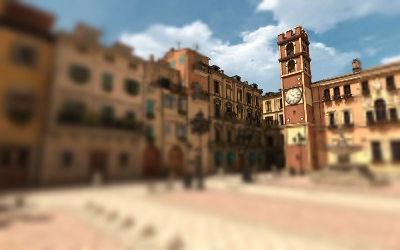Applies a radial tilt shift blur to the image. This effectively creates a circular area in the image that is in focus and the pixels gradually become unfocused (blurred) as they move away from the circle.

 Constructor Description RadialTiltShiftEffect(     radius = 0.3,     gradientSize = 0.3,     blurRadius = 0.0,     blurScale = 1.0,     center = (0.5, 0.5),     downsample = 0.5 ) Create the radial tilt shift effect with the initial parameters.